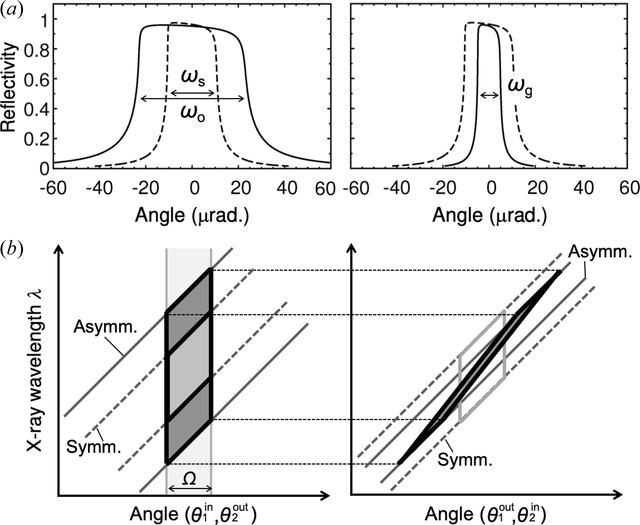disable zoom     view article Figure 2 (a) Rocking curves in (left) the incident condition and (right) the exit condition for the first crystal at 12.4 keV with absorption. α = 6° and b = 0.21 in equation (3), where ωs, ωo and ωg represent the intrinsic Darwin width in the symmetric case (α = 0°), the incident acceptance and the exit reflectance, respectively. (b) DuMond diagrams in both symmetric and asymmetric geometries, where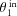and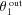represent the incident and exit angles on the first crystal, respectively. The same applies to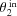and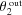on the second crystal.JOURNAL OFSYNCHROTRONRADIATION
ISSN: 1600-5775
Volume 26| Part 3| May 2019| Pages 750-755